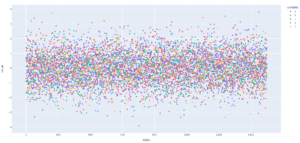# How to change default plotting engine

The default plotting engine that handles plots in Pandas is Matplotlib. I will show you how to change default plotting engine in Pandas.

Matplotlib is a very good library and it displays plots correctly in Python. However, if you want to change Matplotlib to another library, Pandas makes it very easy to change the backend.

## How to change default plotting engine

Just use this command:

```pd.options.plotting.backend = 'plotly'

```

This command will change the default engine from Matplotlib to Plotly.

An example code to generate a chart using Plotly as an engine is as below.

```import pandas as pd
import numpy as np

my_data = np.random.normal(size=[1500, 5])
my_df = pd.DataFrame(my_data)

pd.options.plotting.backend = 'plotly'

my_plot = my_df.plot(kind='scatter')
my_plot.show()

```

Note that in order to use the plotly backend, you’ll need to have plotly installed in your environment.The backends you can use are:

• matplotlib
• plotly
• hvplot
• holoviews
• bokeh
• altair

## Alternative solution for a single chart

You can also use another command that will change the plotting backend for only one plot.

```my_df.plot(backend='plotly')

```

Check plot function documentation to get to know more.